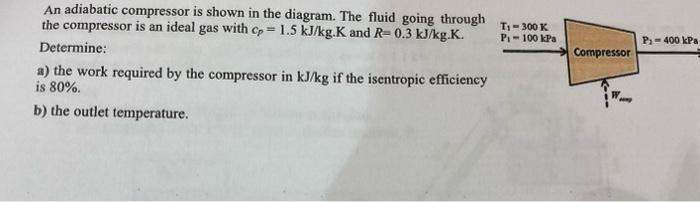# (Solved): An adiabatic compressor is shown in the diagram. The fluid going through the compressor is an ideal ...An adiabatic compressor is shown in the diagram. The fluid going through the compressor is an ideal gas with $$c_{p}=1.5 \mathrm{~kJ} / \mathrm{kg} \cdot \mathrm{K}$$ and $$R=0.3 \mathrm{~kJ} / \mathrm{kg} \cdot \mathrm{K}$$. Determine: a) the work required by the compressor in $$\mathrm{kJ} / \mathrm{kg}$$ if the isentropic efficiency is $$80 \%$$. b) the outlet temperature.

We have an Answer from Expert